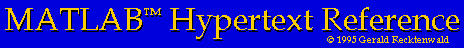# Scalar Variables

## Scalars

In MATLAB a scalar is a variable with one row and one column. Scalars are the simple variables that we use and manipulate in simple algebraic equations.

### Creating scalars

To create a scalar you simply introduce it on the left hand side of an equal sign.
```	>> x = 1;
>> y = 2;
>> z = x + y;
```

### Scalar operations

MATLAB supports the standard scalar operations using an obvious notation. The following statements demonstrate scalar addition, subtraction, multiplication and division.
```	>> u = 5;
>> v = 3;
>> w = u + v
>> x = u - v
>> y = u * v
>> z = u/v
```